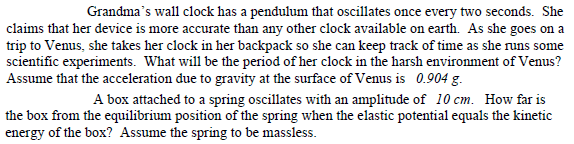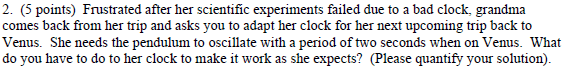# Pendulum - period and length

reminiscent

## Homework StatementT=2pi√(L/g)

## The Attempt at a Solution

I am just making sure I am doing these right.
For the first one, I used the equation to determine the length it would be on Earth. The length I got was g/pi^2 = 0.993 m. I thought that it would have to stay the same length on Venus too, so I used the equation again, given the length and gravity of Venus, to find the period. My answer: 2.10 seconds.
For the second one, I used the equation again, plugged in 2 seconds for T, 0.904g for g, then found L = 0.898 m. I subtracted this from the original length I found from the first problem. My answer: 0.993-0.898 m = 0.095 m (shorten the pendulum by 0.095 m).

drvrm
For the second one, I used the equation again, plugged in 2 seconds for T, 0.904g for g, then found L = 0.898 m. I subtracted this from the original length I found from the first problem. My answer: 0.993-0.898 m = 0.095 m (shorten the pendulum by 0.095 m).

if i am correct the 2nd problem seems to be decoupled from the 'Gandma 's clock' as its a spring oscillator and springs do not oscillate as simple hanging bob and its time period has other parameters.... like spring constant and mass. so think about the second one in a different way !

Homework Helper
Gold Member
OK, for the first question. However, a nice way to approach this problem is in terms of ratios. If you set up an expression for the ratio of the period on Venus to the period on earth, you won't need to find the length.

Your answer to the second question doesn't appear to correspond to the second question.

•drvrm
reminiscent
OK, for the first question. However, a nice way to approach this problem is in terms of ratios. If you set up an expression for the ratio of the period on Venus to the period on earth, you won't need to find the length.

Your answer to the second question doesn't appear to correspond to the second question.
I see what you are saying for the first one. However, for the second one, all I did was find the length the pendulum has to be in order to get a period of 2 seconds. I found that length, and I subtracted it from the length it was on Earth, and my answer is that she would have to shorten it by 0.095 m. That makes sense to me.

drvrm
I see what you are saying for the first one. However, for the second one, all I did was find the length the pendulum has to be in order to get a period of 2 seconds. I found that length, and I subtracted it from the length it was on Earth, and my answer is that she would have to shorten it by 0.095 m. That makes sense to me.